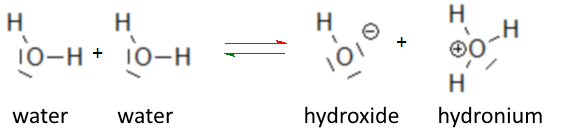Search:

# Chemical Species in pure water

From the autoprotolysis of water equilibrium:we deduce that - pure water contains three chemical species, namely $H_2O$ water, hydroxide ion $OH^-$ and the hydronium ion (hydroxonium, oxonium) $H_3O^+$ - hydroxide and hydronium must be equal in concentration, because each time it appears or disappears an hydroxide ion, it appears or disappears an hydronium ion.

Conductivity measurements showed that in pure water we have:

At 25oC: $[OH-]$ $=$ $[H_3O^+]$ $=$ $10^{-7}\frac{mol}{L}$

We calculated (assuming pure water was only undissociated as $H_2O$) at 25oC the formal concentration of pure water: $c_{H_2O}$ $=$ $55.36 \frac{mol}{L}$ Obviously the $10^{-7}$ mol per liter which dissociate do not cause great changes and it can be argued that even the real concentration of pure water is:

At 25oC: $[H_2O]= 55.36\frac{mol}{L}$